3. Sort

3.1 Bubble Sort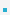Bubble sort is a simple sorting algorithm. Although the algorithm is simple, it is not efficient for    sorting large lists; other algorithms are better.Analysis: O(n2)

 void bubble_sort(int list[], int n) {         int i, j;         for (i=0; ii; j--)                         if (list[j-1]>list[j])                                 swap(&list[j-1], &list[j]);         } }

3.2 Insertion SortAlgorithm
– Every repetition of insertion sort removes an element from the input data, inserting it into the    correct position in the already-sorted list, until no input elements remain.
– In each iteration the first remaining entry of the input is removed, inserted into the result at the    correct position, thus extending the result: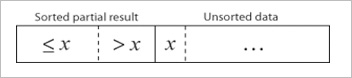becomes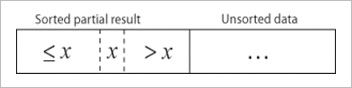void insertion_sort(int list[], int n) {          int i, j;          int temp;          for (j=1; j=0 && list[i]>temp) {                           list[i+1] = list[i];                           i--;                  }                  list[i+1] = temp;          } }

j
     
-
 5 4 3 2 1
1
 4 5 3 2 1
2
 3 4 5 2 1
3
 2 3 4 5 1
4
 1 2 3 4 5Analysis: O(n2)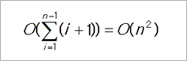3.3 Quick SortQuick sort is a sorting algorithm developed by Tony Hoare that, on average.Analysis: In the average O(n log n) and in the worst case, O(n2).
① Quick sort is often faster in practice than other O(n log n) algorithms.Algorithm
- The steps are:
① When n<=1, the list is sorted.
When n>1, select a pivot element from out of n elements.
② Partition the n elements into 3 segments left, middle, and right.
The middle segment contains only the pivot element.
All elements in the left segments are <= pivot.
All elements in the right segments are >= pivot.
③ Sort left and right segments recursively.
- Answer is sorted left segment, followed by middle segment followed by sorted right segment.

Examplelist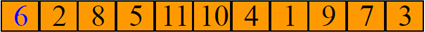① Select a pivot as list[left] = 6
② Partition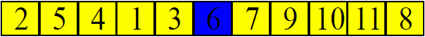③ Sort left and right segments recursively.

Choice of pivotLeft most element
– Pivot is left most element in list that is to be sorted.
– When sorting list[6:20], use list as the pivot.Random selection§
– Randomly select any one such that left <= pivot <= right
– When sorting list[6:20], generate a random number r in the range [6, 20]. Use list[r] as the pivot.Median-of-three rule
– Select the one with median key as the pivot.
– When sorting list[6:20], examine list, list[(6+20)/2], and list. Select the element with median    (i.e., middle) key.
– If list = 30, list = 2, and list = 10, list becomes the pivot.
– If list = 3, list = 2, and list = 10, list becomes the pivot.

C code (version 1)
 void quick_sort(int list[], int left, int right) {     int pivot, i, j;     if (left < right) {         // select a pivot         pivot = list[left];         // partition         i = left;         j = right+1;         do {             do i++; while (list[i] < pivot);             do j--; while (list[j] > pivot);             if (i < j) swap(&list[i], &list[j]);         } while (i < j);         swap(&list[left], &list[j]);         // sort left and right segments recursively         quick_sort(list, left, j-1);         quick_sort(list, j+1, right);     } }

C code (version 2)
 void quick_sort(int list[], int left, int right) {     int pivot, i, mid;     if (left < right) {         // pivot is midpoint; move to left side         swap(&list[left], &list[(left+right)/2]);         pivot = list[mid=left];         // partition         // left side < pivot (left+1 to mid),         // right side >= pivot (mid+1 to right)         for (i=left+1; i<=right; i++) {             if (list[i] < pivot)                 swap(&list[i], &list[++mid]);         }         // resotre pivot position         swap(&list[left], &list[mid]);         if (mid > left) quick_sort(list, left, mid-1);         if (mid < right) quick_sort(list, mid+1, right);     } }

Average ComplexityIf T(n) is the time taken to sort a list of n records, then when the list splits roughly into two equal parts   each time a record is positioned correctly, we have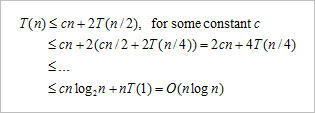3.4 Comparison of Sort Methods

 Name Best Average Worst Bubble sort n n2 n2 Insertion sort n n2 n2 Quick sort n log n n log n n2 Merge sort n log n n log n n log n Heap sort n log n n log n n log n

 이전페이지 / 4 / 다음페이지